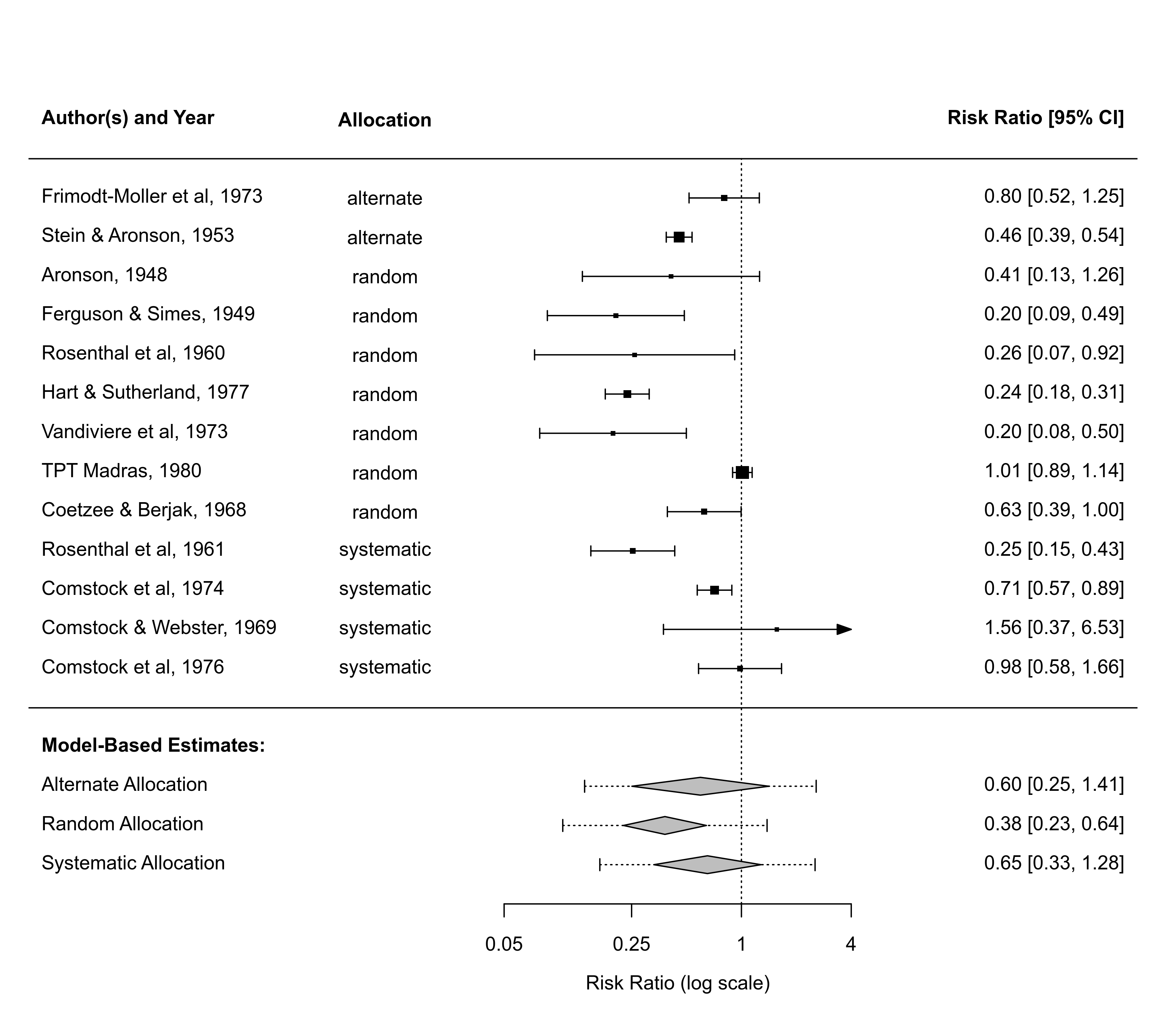Function to add one or more polygons to a forest plot based on an object of class "predict.rma".

# S3 method for predict.rma
addpred=FALSE, digits, width, mlab, transf, atransf, targs,
efac, col, border, lty, fonts, cex, ...)

## Arguments

x

an object of class "predict.rma".

rows

vector to specify the rows (or more generally, the horizontal positions) for plotting the polygons (defaults is -2). Can also be a single value to specify the row (horizontal position) of the first polygon (the remaining polygons are then plotted below this starting row).

annotate

optional logical to specify whether annotations should be added to the plot for the polygons that are drawn.

logical to specify whether the bounds of the prediction interval should be added to the plot (the default is FALSE).

digits

optional integer to specify the number of decimal places to which the annotations should be rounded.

width

optional integer to manually adjust the width of the columns for the annotations.

mlab

optional character vector with the same length as x giving labels for the polygons that are drawn.

transf

optional argument to specify a function to transform the x values and confidence interval bounds (e.g., transf=exp; see also transf).

atransf

optional argument to specify a function to transform the annotations (e.g., atransf=exp; see also transf).

targs

optional arguments needed by the function specified via transf or atransf.

efac

optional vertical expansion factor for the polygons.

col

optional character string to specify the color to use for the polygons. If unspecified, the function sets a default color.

border

optional character string to specify color to use for the border of the polygons. If unspecified, the function sets a default color.

lty

optional character string to specify the line type for the prediction interval. If unspecified, the function sets this to "dotted" by default.

fonts

optional character string to specify the font to use for the labels and annotations.

cex

optional symbol expansion factor.

...

other arguments.

## Details

The function can be used to add one or more polygons to an existing forest plot created with the forest function. For example, summary estimates based on a model involving moderators can be added to the plot this way (see ‘Examples’).

To use the function, one should specify the values at which the polygons should be drawn (via the x argument) together with the corresponding variances (via the vi argument) or with the corresponding standard errors (via the sei argument). Alternatively, one can specify the values at which the polygons should be drawn together with the corresponding confidence interval bounds (via the ci.lb and ci.ub arguments). Optionally, one can also specify the bounds of the corresponding prediction interval bounds via the pi.lb and pi.ub arguments.

If unspecified, arguments annotate, digits, width, transf, atransf, targs, efac (only if the forest plot was created with forest.rma), fonts, cex, annosym, and textpos are automatically set equal to the same values that were used when creating the forest plot.

## Author

Wolfgang Viechtbauer wvb@metafor-project.org https://www.metafor-project.org

forest.rma and forest.default for functions to draw forest plots to which polygons can be added.

## Examples

### calculate log risk ratios and corresponding sampling variances
dat <- escalc(measure="RR", ai=tpos, bi=tneg, ci=cpos, di=cneg,
data=dat.bcg, slab=paste(author, year, sep=", "))

### forest plot of the observed risk ratios
with(dat, forest(yi, vi, atransf=exp, xlim=c(-8,4), ylim=c(-4.5,16),
at=log(c(.05, .25, 1, 4)), cex=.8, order=alloc,

### fit mixed-effects model with allocation method as a moderator
res <- rma(yi, vi, mods = ~ 0 + alloc, data=dat)

### predicted log risk ratios for the different allocation methods
x <- predict(res, newmods=diag(3))

### add predicted average risk ratios to forest plot